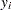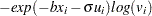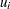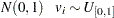The MODEL Procedure

## Example 18.18 Duration Data Model with Unobserved Heterogeneity

All of the previous three models actually have closed-form moment conditions, so the simulation approach is not necessarily required for the estimation. This example illustrates how to use SMM to estimate a model for which there is no closed-form solution for the moments and thus the traditional GMM method does not apply. The model is the duration data model with unobserved heterogeneity in Gourieroux and Monfort (1993):The SAS statements are:

```
title1 'SMM for Duration Model with Unobserved Heterogeneity';

%let nobs=1000;
data durationdata;
b=0.9; s=0.5;
do i=1 to &nobs;
u = rannor( 1011 );
v = ranuni( 1011 );
x = 2 * ranuni( 1011 );
y = -exp(-b * x + s * u) * log(v);
output;
end;
run;

proc model data=durationdata;
parms b .5 s 1;
instrument x;

u = rannor( 1011 );
v = ranuni( 1011 );
y = -exp(-b * x + s * u) * log(v);

moment y = (2 3 4);
fit y / gmm ndraw=10 ;* maxiter=500;
bound s > 0, b > 0;
run;
```

The output of the MODEL procedure is shown in Output 18.18.1.

Output 18.18.1 PROC MODEL Output
 SMM for Duration Model with Unobserved Heterogeneity

The MODEL Procedure

Model Summary
Model Variables 1
Parameters 2
Equations 4
Number of Statements 9

Model Variables y b(0.5) s(1) _moment_3 _moment_2 _moment_1 y

The 4 Equations to Estimate
_moment_3 = F(b, s)
_moment_2 = F(b, s)
_moment_1 = F(b, s)
y = F(b, s)
Instruments 1 x

Nonlinear GMM Parameter Estimates
Parameter Estimate Approx Std Err t Value Approx
Pr > |t|
b 0.92983 0.0331 28.08 <.0001
s 0.341825 0.0608 5.62 <.0001Previous Page | Next Page | Top of Page